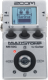# proba

Discussion in 'Zoom MS-50G' started by Kronicek, Jun 4, 2019.

1. probaDevice: Zoom Ms-50g
Firmware: 3.00

Name on device: Fender
Optimized for: Guitar Amp

Effects chain:kkk

Effect: "ZNR" (Dynamics / Filter), active - "yes"
"THRSH" = 12
"DETCT" = GtrIn
"Level" = 100

Effect: "Squeak" (Overdrive / Distortion), active - "yes"
"Gain" = 3
"Tone" = 75
"Level" = 8

Effect: "VintageCE" (Modulation / Sfx), active - "yes"
"Comp" = 3
"Rate" = 18
"Mix" = 8
"Level" = 150

Effect: "ParaEQ" (Dynamics / Filter), active - "yes"
"Freq1" = 63Hz
"Q1" = 0.5
"Gain1" = 6
"Freq2" = 4.0kHz
"Q2" = 0.5
"Gain2" = 3
"Level" = 150

Effect: "ParaEQ" (Dynamics / Filter), active - "yes"
"Freq1" = 400Hz
"Q1" = 0.5
"Gain1" = 1
"Freq2" = 630Hz
"Q2" = 0.5
"Gain2" = -3
"Level" = 100

Effect: "ParaEQ" (Dynamics / Filter), active - "yes"
"Freq1" = 250Hz
"Q1" = 0.5
"Gain1" = 6
"Freq2" = 250Hz
"Q2" = 0.5
"Gain2" = 4
"Level" = 100

Note: This is a patch file, you will need to download and install the ToneLib-Zoom software to use the patch.

File size:
406 bytes
Views:
100